# CHEMICAL EQUATIONS Chapter 9 CHEMICAL REACTIONS A chemical

• Slides: 20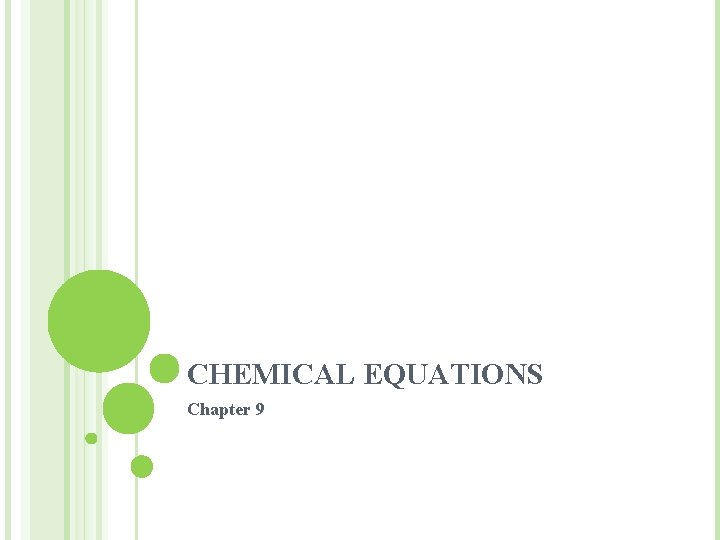CHEMICAL EQUATIONS Chapter 9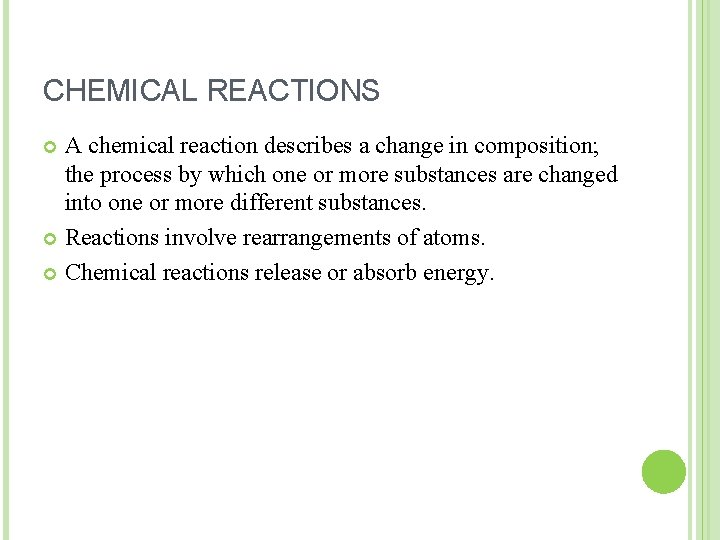CHEMICAL REACTIONS A chemical reaction describes a change in composition; the process by which one or more substances are changed into one or more different substances. Reactions involve rearrangements of atoms. Chemical reactions release or absorb energy.CHEMICAL EQUATION An expression showing the formulas and the relative amounts of the reactants and products in a chemical reaction. A chemical equation describes the type and number of atoms that are rearranged during a reaction.TIPS FOR BALANCING EQUATION Balancing chemical equations literally means counting the number of times atom symbols appear in the reactants and products to make sure the counts are the same on both sides. Conservation of mass is linked to "conservation " of element symbols. The law of conservation of mass is met when the count of element symbols on reactants side is equal to the count of element symbols on the product side. balanced equation has equal counts (number) of atoms of each element in both reactants and products. Equations are balanced by adjusting the multipliers (coefficients) in front of formula symbols so the counts of atoms are the same in reactants and products. The subscripts are not changed.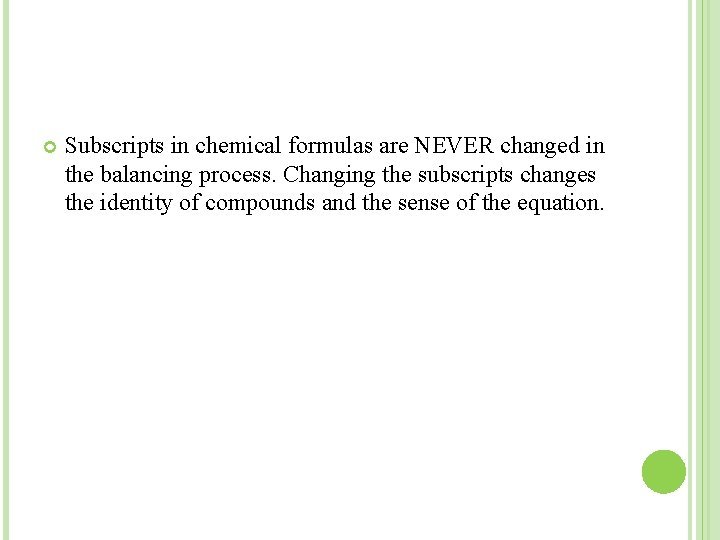Subscripts in chemical formulas are NEVER changed in the balancing process. Changing the subscripts changes the identity of compounds and the sense of the equation.CLASS PRACTICE Balance the following equations: Page 316 Q. 1. a, b, c, dQUANTITATIVE RELATIONSHIP Equations reveal the number of formula units involved. The coefficients from a balanced equation can help you to predict how much of each reactant is needed to make a certain amount of product. C 6 H 12 O 6(aq) +6 O 2(g) 6 CO 2(g) +6 H 2 O(l) +energy In this equation we can express as moles, formula units or molecules. Mass is conserved and atoms are conserved. Total number of moles and molecules do not necessarily remain the same on both sides of the chemical equation since molecules are created and destroyed as atoms are rearranged.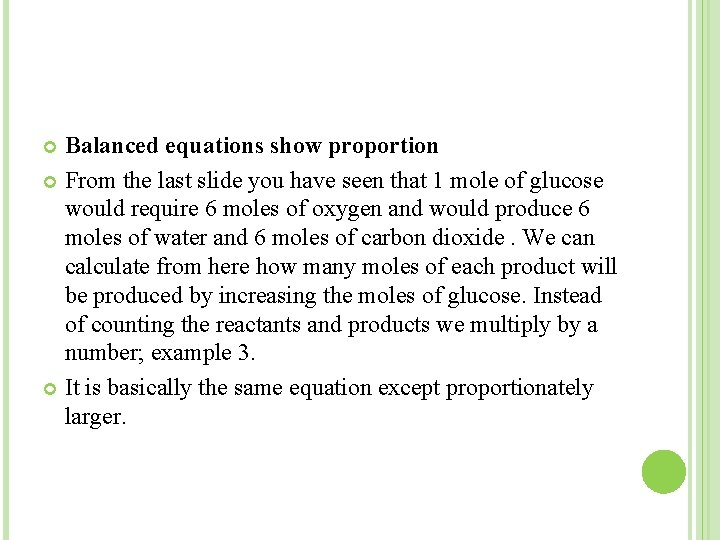Balanced equations show proportion From the last slide you have seen that 1 mole of glucose would require 6 moles of oxygen and would produce 6 moles of water and 6 moles of carbon dioxide. We can calculate from here how many moles of each product will be produced by increasing the moles of glucose. Instead of counting the reactants and products we multiply by a number; example 3. It is basically the same equation except proportionately larger.Relative amounts in equations can be expressed in moles. The molar interpretation of the previous reaction can be expressed as ratios of reactants and products. 1 mol C 6 H 12 O 6: 6 mol O₂ : 6 mol CO₂ : 6 mol H₂O For any pair of molecules or formula units, a mole ratio can be determined by comparing the coefficients from the balanced chemical equation. Usually expressed as lowest whole number ratios.EXOTHERMIC AND ENDOTHERMIC REACTIONS The amount of energy released or absorbed in a chemical reaction is expressed as ∆H, where ∆ stands for “change in”, and H represents enthalpy, the total energy content of a system. ∆H values are negative because energy is released when the stronger bonds of the products are created. In endothermic reactions energy is added to the system and ∆H values are positive because the energy needed to break the bonds increases the total energy of the system.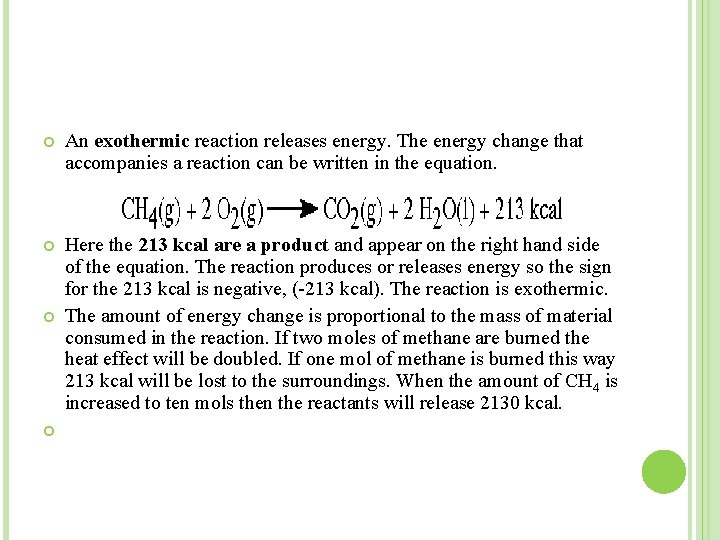An exothermic reaction releases energy. The energy change that accompanies a reaction can be written in the equation. Here the 213 kcal are a product and appear on the right hand side of the equation. The reaction produces or releases energy so the sign for the 213 kcal is negative, (-213 kcal). The reaction is exothermic. The amount of energy change is proportional to the mass of material consumed in the reaction. If two moles of methane are burned the heat effect will be doubled. If one mol of methane is burned this way 213 kcal will be lost to the surroundings. When the amount of CH 4 is increased to ten mols then the reactants will release 2130 kcal.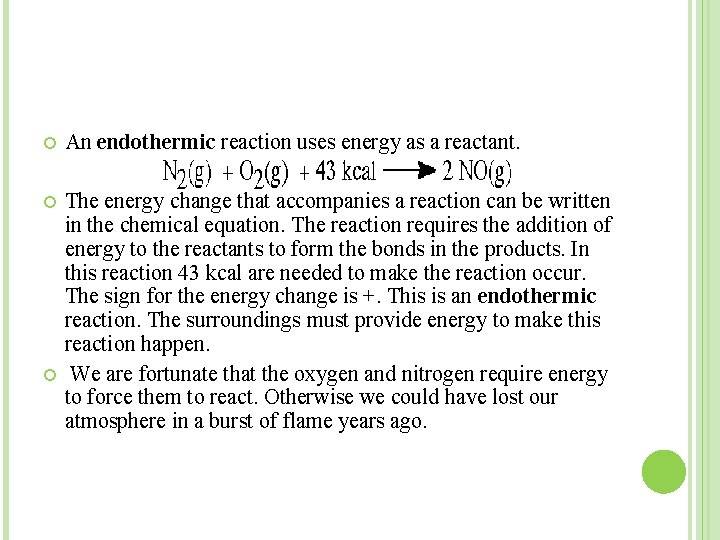An endothermic reaction uses energy as a reactant. The energy change that accompanies a reaction can be written in the chemical equation. The reaction requires the addition of energy to the reactants to form the bonds in the products. In this reaction 43 kcal are needed to make the reaction occur. The sign for the energy change is +. This is an endothermic reaction. The surroundings must provide energy to make this reaction happen. We are fortunate that the oxygen and nitrogen require energy to force them to react. Otherwise we could have lost our atmosphere in a burst of flame years ago.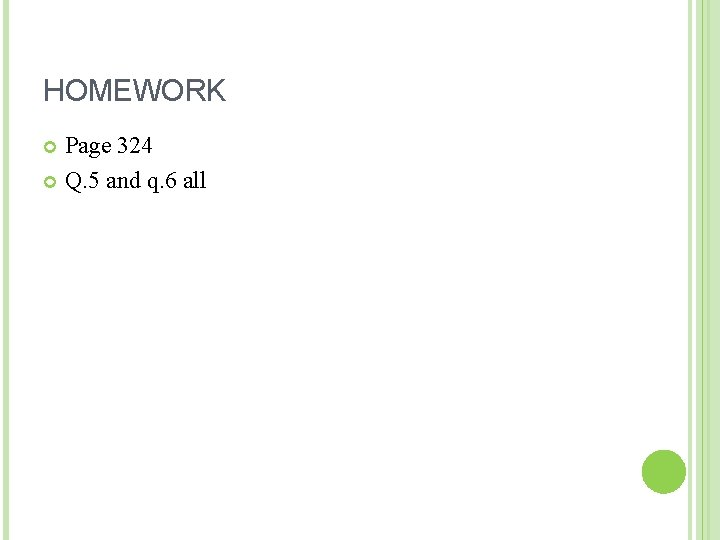HOMEWORK Page 324 Q. 5 and q. 6 allCLASSIFICATION OF CHEMICAL REACTIONS There are several types of chemical reactions: Combustion: A combustion reaction is when oxygen combines with another compound to form water and carbon dioxide. These reactions are exothermic, meaning they produce heat. An example of this kind of reaction is the burning of napthalene: C 10 H 8 + 12 O 2 ---> 10 CO 2 + 4 H 2 OSynthesis: A synthesis reaction is when two or more simple compounds combine to form a more complicated one. These reactions come in the general form of: A + B ---> AB One example of a synthesis reaction is the combination of iron and sulfur to form iron (II) sulfide: 8 Fe + S 8 ---> 8 Fe. S Below a skinny bird(reactant) and a worm(reactant) combine to form a fat bird.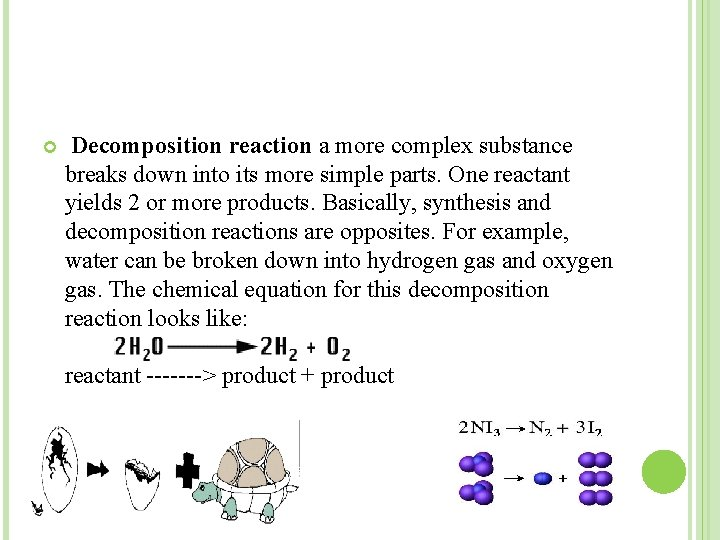Decomposition reaction a more complex substance breaks down into its more simple parts. One reactant yields 2 or more products. Basically, synthesis and decomposition reactions are opposites. For example, water can be broken down into hydrogen gas and oxygen gas. The chemical equation for this decomposition reaction looks like: reactant -------> product + product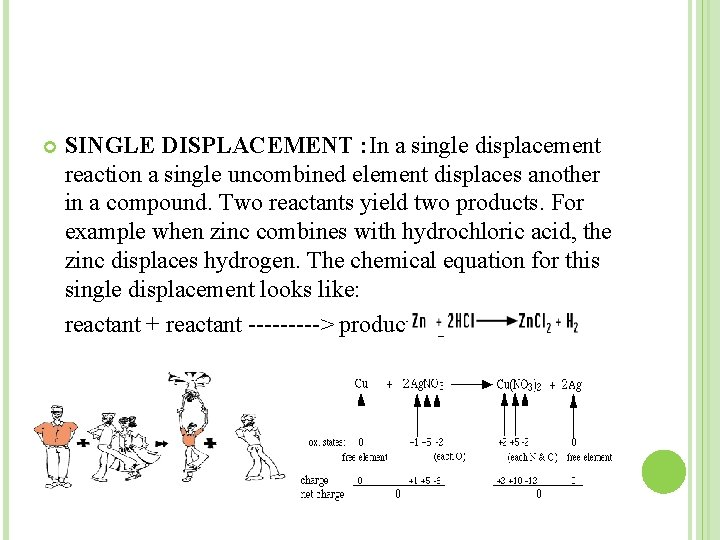SINGLE DISPLACEMENT : In a single displacement reaction a single uncombined element displaces another in a compound. Two reactants yield two products. For example when zinc combines with hydrochloric acid, the zinc displaces hydrogen. The chemical equation for this single displacement looks like: reactant + reactant -----> product + productDOUBLE DISPLACEMENT : In a double displacement reaction parts of two compounds switch places to form two new compounds. Two reactants yield two products. For example when silver nitrate combines with sodium chloride, two new compounds--silver chloride and sodium nitrate are formed because the sodium and silver switched places. The chemical equation for this double displacement reaction looks like: reactant + reactant -----> product + product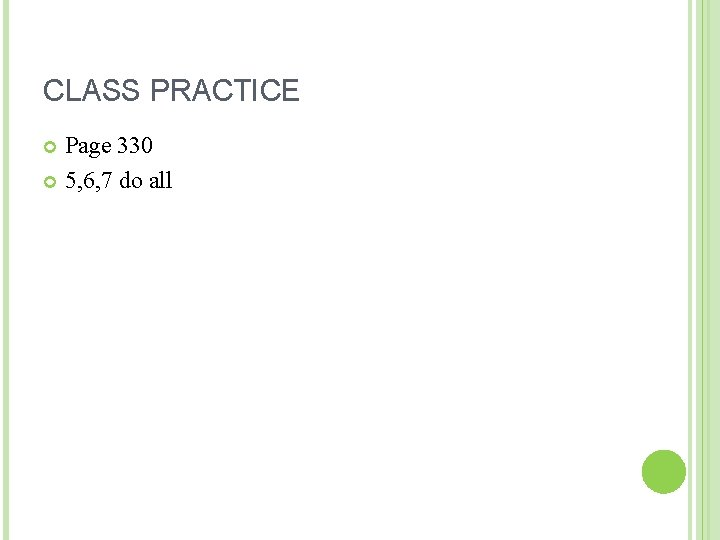CLASS PRACTICE Page 330 5, 6, 7 do allHOMEWORK Q. 28, 29 do all Q. 32 ; a and b Q. 43 ; a, b and d.